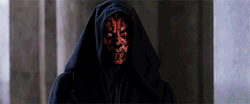t

How to expand a circle to your viewport

At the time of writing, my homepage features a neat little transition where a circle grows from the center of the page as you scroll down until it fills the screen. The next section starts immediately after the circle fills the entirety of the viewport.

It's like the inverse of this moment from The Phantom Menace:This post is going to explain how I achieved this effect and the mathematical principles that make it possible.

The first thing I need to do is determine what size circle is needed to fill the viewport. Or put another way, what is the diameter of a circle that will snugly fit the viewport within it?

The diameter of the circle will depend on the size of the viewport. As the viewport grows or shrinks in either dimension, so too should the circle's diameter. The diameter of the circle, marked as $d$ in the diagram below, is the same as the hypotenuse of the right triangle created by slicing the viewport in half diagonally.

Using the Pythagorean theorem, we can calculate the length of the hypotenuse using the width and height of the viewport.

\begin{aligned} a^2 + b^2 &= c^2 \\ w^2 + h^2 &= d^2 \\ d &= \sqrt{w^2 + h^2} \end{aligned}

I implemented this technique in React with the help of the handy useWindowSize hook from react-use. As its name implies, this hook tracks the dimensions of the browser window. With this information, I can calculate the diameter of the circle and render it in the page.

import {useWindowSize} from 'react-use';
function MyComponent() {
const {width, height} = useWindowSize();
const diameter = Math.sqrt(width ** 2 + height ** 2);
return (
<div
style={{
width: diameter,
height: diameter,
backgroundColor: 'black',
borderRadius: '50%', // make it round
// absolutely position the circle in the center of the page
position: 'absolute',
top: '50%',
left: '50%',
transform: 'translate(-50%, -50%)'
}}
/>
);
}

The big wipe

To complete the effect, I synchronized the growth of the circle with the page's scroll position using a CSS scale transform. I calculated the scale amount by dividing the current scroll position by the height of the window, and clamping it.

Clamping is the process of limiting a value to a specific range. In this case, I want the scale to be a number between or including 1 and 0. In JavaScript, a number can be clamped using the following snippet:

const clamped = Math.min(max, Math.min(min, number));

💡 Did you know?

useWindowScroll is another handy hook from the react-use that tracks the scroll position.

import {useWindowScroll, useWindowSize} from 'react-use';
function MyComponent() {
const {y} = useWindowScroll();
const {width, height} = useWindowSize();
// calculate diameter
const scale = Math.min(1, Math.max(0, y) / height);
return (
<div
style={{
// other styles
transform: translate(-50%, -50%) scale(\${scale})
}}
/>
);
}

In this example, the scale amount will start at 0 (invisible) when the scrollbar is at the top of the page, and reach 1 (full size) after one height of the viewport has been scrolled.

To complete the effect, the circle must follow the user as they scroll down the page and then lock into place before scrolling out of view when the next section comes into frame. This is where sticky positioning really shines.

I wrap my circle in a sticky-positioned container with a height of one viewport or 100vh, and then wrap that in another container that is 200vh high. The outer container acts as a "track" for the first section's content and circle. It ensures that after one viewport height is scrolled, there is enough room left over for the fully scaled circle to occupy its own one viewport of height.

Check out the CodeSandbox below for a complete example and live demo of this effect in action.

Resources

As I was writing this post, I realized that Google used a similar technique on their Pixel 5 website. If you end up doing something like this in your own website, I'd be thrilled if you let me know!

I leaned on a few helpful resources to understand and execute this feature. If you want to learn more about the mathematical techniques highlighted in this post, I suggest checking them out:

Made with 🥥 in Burnaby, BC